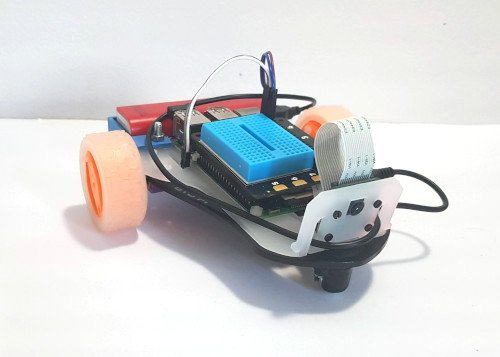# STS-Pi Raspberry Pi Robot

This is the STS-Pi robot from Pimoroni. It comes with a chassis, two motors and wheels, an omnidirectional wheel and camera mount. You need to add your own Raspberry Pi and battery. You also need a motor controller which they recommend an Explorer HAT, Explorer HAT Pro or Explorer pHAT.

It comes with a battery holder for a cylindrical battery, but it did not fit the battery I had. I have therefore created a battery holder designed in FreeCAD and then 3D printed.The code used in the demo is designed for control using a keyboard. You will need to launch the application first and ensure it has focus which can be achieved using an external screen or VNC.

```
```

#!/usr/bin/python3

import sys, tty, termios

import picamera, time

from gpiozero import Robot

# Pin numbres

# CamJam 10,9 - 8,7

# STS-Pi 19,20 - 21,26

robot = Robot(left=(19, 20), right=(21, 26))

camera_enable = False

try:

camera = picamera.PiCamera()

camera.hflip=True

camera.vflip=True

camera_enable=True

except:

photo_dir = "/home/pi/photos"

# get a character from the command line

def getch() :

fd = sys.stdin.fileno()

old_settings = termios.tcgetattr(fd)

try:

tty.setraw(sys.stdin.fileno())

finally:

return ch

# list to convert key presses into motor on/off values to correspond with the direction

# direction based on number keypad

# 8 = fwd, 4 = left, 5 = stop, 6 = right, 2 = rev

# the key for the list is the character

direction = {

# number keys

'2' : "backward",

'4' : "left",

'5' : "stop",

'6' : "right",

'8' : "forward",

'w' : "forward", 'a' : "left", 'd' : "right", 's' : "stop", 'x' : "backward"

}

current_direction = "stop"

# speed is as a percentage (ie. 100 = top speed)

# start speed is 50% which is fairly slow on a flat surface

speed = 50

print ("Robot control - use number keys to control direction")

print ("Speed " + str(speed) +"% - use +/- to change speed")

while True:

# Convert speed from percentage to float (0 to 1)

float_speed = speed / 100

if (current_direction == "forward") :

robot.forward(float_speed)

# rev

elif (current_direction == "backward") :

robot.backward(float_speed)

elif (current_direction == "left") :

robot.left(float_speed)

elif (current_direction == "right") :

robot.right(float_speed)

# stop

else :

robot.stop()

# Get next key pressed

ch = getch()

# q = quit

if (ch == 'q') :

break

elif (ch == '+') :

speed += 10

if speed > 100 :

speed = 100

print ("Speed : "+str(speed))

elif (ch == '-' ) :

speed -= 10

if speed < 0 :

speed = 0

print ("Speed : "+str(speed))

elif (ch in direction.keys()) :

current_direction = direction[ch]

print ("Direction "+current_direction)

elif (ch == '0' and camera_enable == True) :

timestring = time.strftime("%Y-%m-%dT%H.%M,%S", time.gmtime())

print ("Taking photo " +timestring)

camera.capture(photo_dir+'/photo_'+timestring+'.jpg')

robot.close()

```

```

## 3D printed battery holder

This can be 3D printed and increases the choice of different batteries which can be attached to the robot. It is recommended this is attached using M4 metal screws (rather than the nylon M5 screws which are used for the supplied bracket).